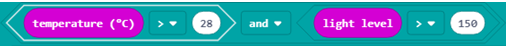# Obeleži sve kategorije koje odgovaraju problemu

### Još detalja - opišite nam problem

Uspešno ste prijavili problem!
Status problema i sve dodatne informacije možete pratiti klikom na link.
Nažalost nismo trenutno u mogućnosti da obradimo vaš zahtev.
Molimo vas da pokušate kasnije.

# Working with Blocks from the Logic Category¶

The programs we created so far were executed block by block, from the first to the last block. This kind of program execution is called sequential. In sequential (linear) execution, skipping (omitting) blocks is not possible. They are all executed once (if they are inside the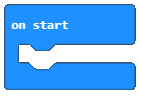block) or multiple times (if they are inside the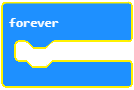block).

However, it may happen that the task you are working on requires that you change the way the program is executed.

For example, if you are creating a program that will be used by younger students to learn computation (arithmetic), you may want to run certain blocks, which you use to commend the correct answer or you may want to run completely different blocks, which provide comments for the incorrect answers.

Based on whether an answer is correct or incorrect, you may have the need to run different sets of blocks. That is why we say that the program forms branches, i.e. that blocks from one branch are being executed, while the blocks from another branch are not.

You make decisions every day and continue your daily activities based on them. For example, the temperature in the classroom is 30 degrees Celsius, and you want to turn on the air conditioner. Your next step would be to check the temperature in the classroom and decide if you want to turn on the air conditioner.

It is similar when it comes to programming.

With regard to decision making, MakeCode offers:

• Comparison operators

• Decision-making blocks (conditional blocks, which form branches within the program)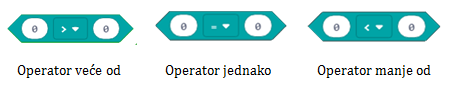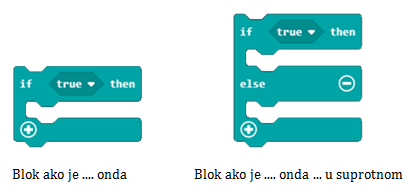By using a comparison operator, you can compare values ​​and determine if one is larger/smaller than the other or if they are equal. The result of the comparison may be TRUE or FALSE.

Q-8: The temperature in the room is 28 degrees Celsius. Analyze the blocks given in the figure and mark the correct statement.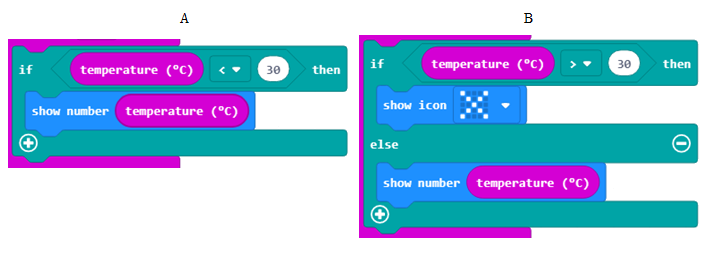• As the result of running the block A, the program will display the value of the temperature in the room.
• The answer is not correct!
• Running the blocks A and B will have the same result - the program will write the room temperature.
• As the result of running the block B, the program will display a little cross.
• The answer is not correct!

Let’s write some programs!

There is a challenge in front of you, if a randomly chosen number (from the interval 0 to 100) is an even number, you should display the text “The number is even.”, and if the number is an odd number, you should display the text “The number is odd.”.

When is a number even, and when is it odd? If by using integer division, we divide a number by 2, and there is no remainder, the number is considered even. If, when we use integer division to divide a number by 2, the remainder is 1, then we know that it is an odd number.

For example, if we use integer division to divide 10 by 2, the result will be 5 because 5*2 equals 10. However, if we divide 9 by 2, we get 4; 4*2 is 8, and we are left with the remainder 1. From here we can conclude that, if there is no remainder when a number is divided by 2 using integer division, then it is an even number. Otherwise, the number is odd.

In MakeCode, you can write integer division by using the operation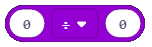, and the remainder by using the block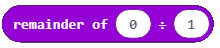, which provides the value for the remainder.

Based on the previous lesson, we will define the variable Number, which stores the value obtained from using the block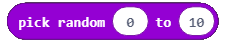, or more specifically, the variable Number will receive one of the random values from the interval from 0 to 100.

The next step would be to check whether there is a remainder in integer division of the Number by 2. If it is true that there isn’t any remainder, then the message “The number is even.” will be displayed. Otherwise, if the condition has not been fulfilled, and there is a remainder, then the message “The number is odd.” will be displayed.

Before we check the condition, we will have to create the variable Remainder, and set a value for the integer division of the Number and number 2: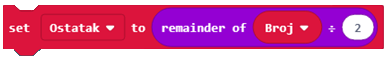Now, the next step would be to check whether the number is even or not. This will be done by introducing the if....then... else block and a comparison operator. If the variable Remainder is 0, the number is even and the message “The number is even.” will be displayed. Otherwise, if the variable Reminder is not 0, the number is odd and the message “The number is odd.” will be displayed.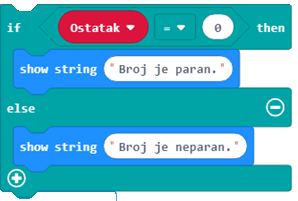The final look of the block: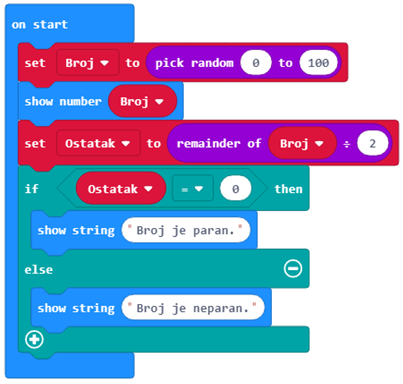You can see the code on the following link: https://makecode.microbit.org/_WDmbuk3kKXW5

To test the program, we will run it in the simulator by clicking on the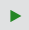button.

Task: Write a program, which simulates rolling dice. If the result of the roll (a movement - shake was registered) is number 1, this number should be represented by one dot; if the result is 2, it should be represented by two dots, and so on.

Compare your solution with ours: https://makecode.microbit.org/_HsxKqAC90d5m

Addition: You can use logical (Boolean) operators for connecting conditions.

In MakeCode, logical operators are presented as follows: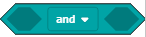- connects two conditions and returns True as the result only if both conditions are True.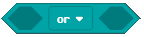- connects two conditions and returns True as the result only if at least one condition is True.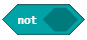- is placed in front of one condition, and returns True as the result in case the condition is not fulfilled.

The condition for checking that the measured temperature is higher than 28, and the light level is higher than 150 (block light level) is checked by this block: